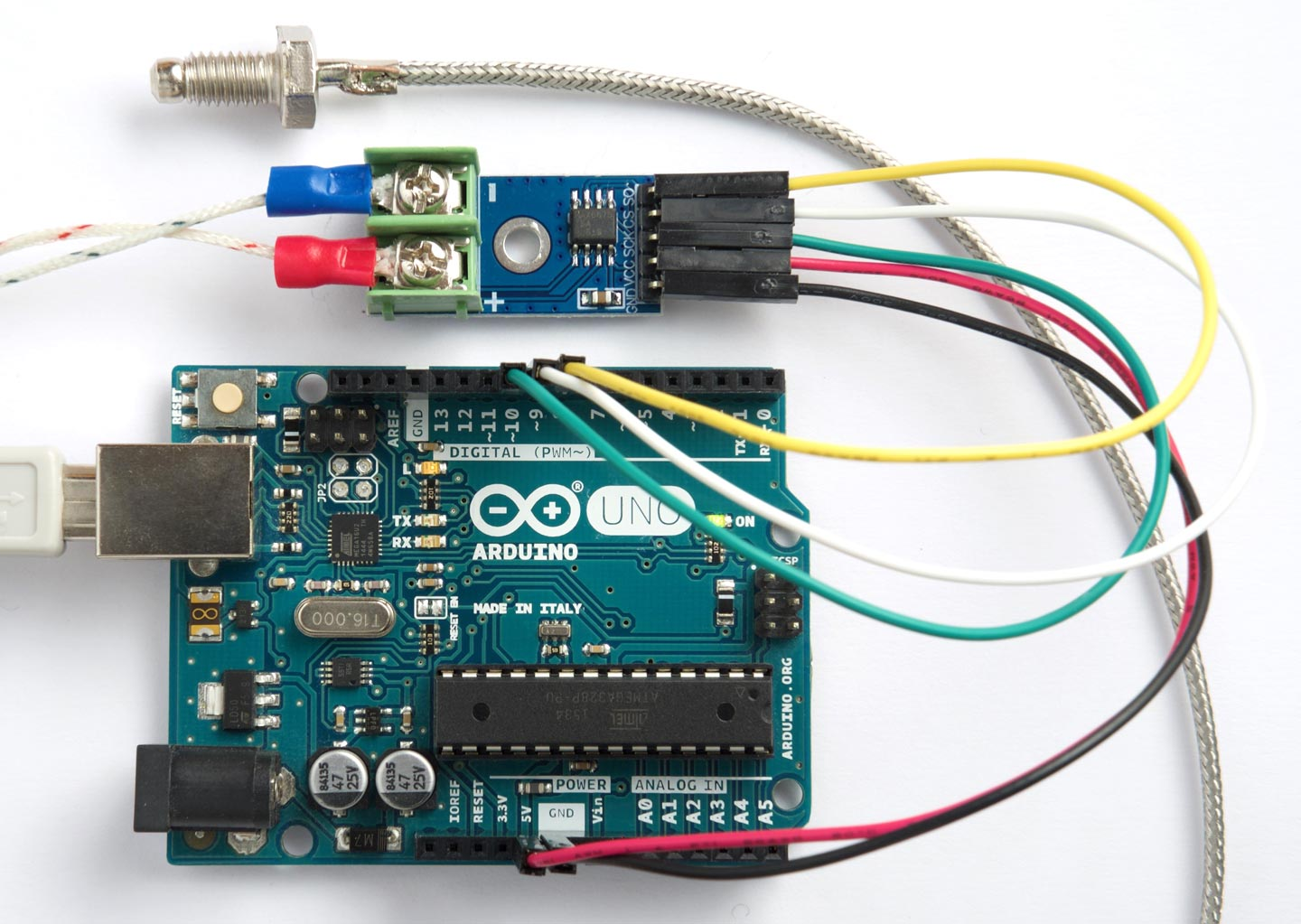## Thermocouple interface

Thermocouples are a great choice for measuring the temperature at a probe, and work over a wide range; for example, the widely-used K-type thermocouple has a range of -200°C to +1350°C.

This post describes a simple thermocouple interface using uLisp, based on a low-cost MAX6675 Thermocouple Board, widely available on eBay and Chinese sites such as Banggood, often with a sensor included :The MAX6675 has a range of 0°C to 1024°C, which is ideal for many applications such as a cooking thermometer, or for measuring soldering temperatures . Although it has been superseded by the MAX31855, which offers a wider temperature range of -270°C to 1800°C, the MAX6675 has the advantages of low cost, and the fact that it will work at 5V, whereas the MAX31855 is 3.3V maximum.

### uLisp interface

The MAX6675 needs to be connected to the Arduino via five cables: 5V, GND, and the three SPI lines SO, CS, and CLK. I used an Arduino Uno, but any board should be suitable.

Here's the uLisp interface:

```(defvar so 8)
(defvar cs 9)
(defvar clk 10)

; Initialise pins
(defun tci ()
(pinmode so nil)
(pinmode cs t)
(pinmode clk t)
(digitalwrite cs 1))

; Read temperature in units of 0.25 degrees C
(defun tco ()
(let ((dat 0))
(digitalwrite cs 0)
(dotimes (i 16)
(digitalwrite clk 1)
(when (digitalread so) (incf dat (ash 1 (- 15 i))))
(digitalwrite clk 0))
(digitalwrite cs 1)
(ash dat -3)))```

The I/O pins used to connect to the MAX6675 are defined by the three defvar statements, so change these if you want to use different pins.

First call (tci) to initialise the I/O pins. Then call (tco) to read the temperature. This returns an integer in units of quarters of a degree Centigrade. For example:

```> (tco)
89```

means that the temperature of the probe is 22.25°C.

What you do with the temperature depends on your application. For example, if you're making a sugar thermometer for sweet-making you could make it display the caramel stage; for example:

```(let ((tmp (/ (tco) 4)))
(cond
((>= 116 tmp 112) "Soft Ball")
...
((>= 130 tmp 121) "Hard Ball"))```

1. ^ MAX6675 Sensor Module Thermocouple on Banggood.
2. ^ MAX6675 datasheet on Maxim Integrated.